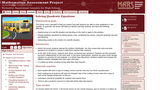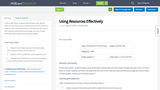# 3 Results

View
Selected filters:
• Math Application
RemixConditional Remix & Share Permitted
CC BY-NC-SA
Rating

This lesson will be an introduction to the many areas where math is utilized in an iron foundry.

Subject:
Mathematics
Material Type:
Lesson
Author:
Lynn Aprill
Monica Wagner
Date Added:
10/10/2019Only Sharing Permitted
CC BY-NC-ND
Rating

This lesson unit is intended to help you assess how well students are able to solve quadratics in one variable. In particular, the lesson will help you identify and help students who have the following difficulties:
Making sense of a real life situation and deciding on the math to apply to the problem.Solving quadratic equations by taking square roots, completing the square, using the quadratic formula, and factoring.Interpreting results in the context of a real life situation.

Subject:
Mathematics
Material Type:
Diagram/Illustration
Formative Assessment
Learning Task
Lesson Plan
Reading
Rubric/Scoring Guide
Self Assessment
Simulation
Provider:
Mathematics Assessment Resource Service
Date Added:
12/15/2016
RemixConditional Remix & Share Permitted
CC BY-NC-SA
Rating

In this math lesson, students will be using math skills to determine the best layout of industrial parts to be cut from a sheet of metal. Students will then calculate both the cost of the materials used and the percentage and cost of waste remaining after cutting out the industrial parts.

Subject:
Career and Technical Education
Mathematics
Material Type:
Lesson
Author:
Nathan Falk
Date Added:
01/16/2020